# Work Equation: Thermodynamics Sample

Problem #1:

Find the amount of the work and the amount of heat transferred when the body is heated to 275 oC, if the volume of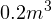is enclosed by a 0.4 kg body at a constant pressure of 0.55 MPa.

Solution:

The kinetic and potential changes of the current system do not significantly change and can be neglected.

Then: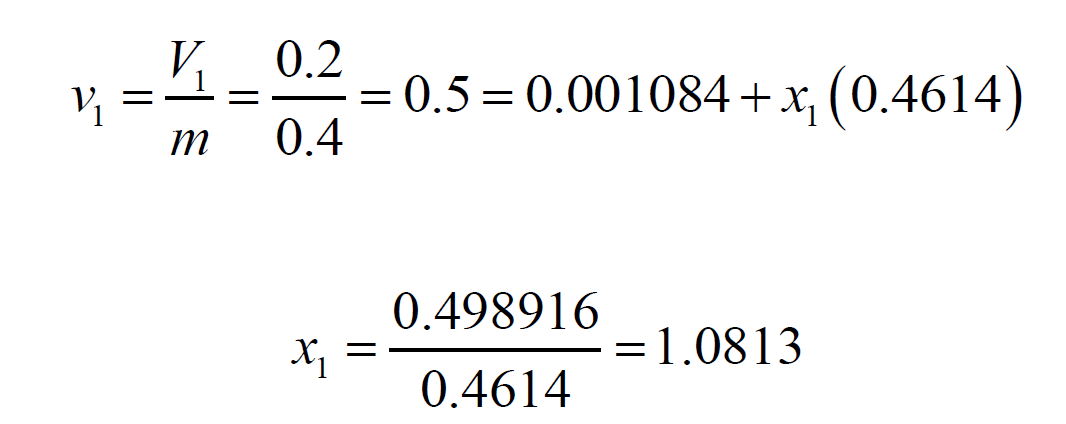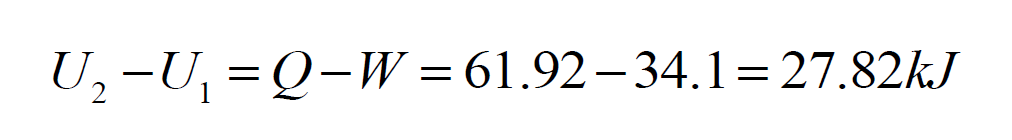The heat transfer can be found then from:

a) Suppose it is required to increase the temperature from -20 to 100 degrees Celsius for 1 kg of nitrogen at a constant pressure. Calculate the required amount of heat.

b) Calculate the increment of the nitrogen’s internal energy.

c) Calculate the required external work.
d) Assuming the volume is constant, calculate the required heat if: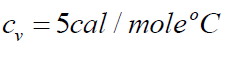and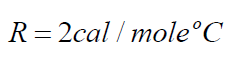Solution:

The kinetic and potential:

a) The nitrogen can be assumed to be an ideal gas. Thus the required heat can be calculated by the following formula: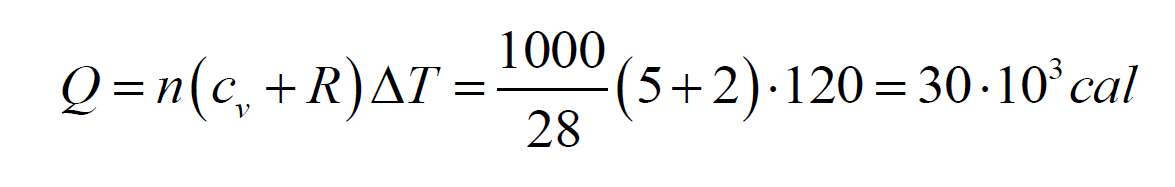b) The increment of the nitrogen’s internal energy: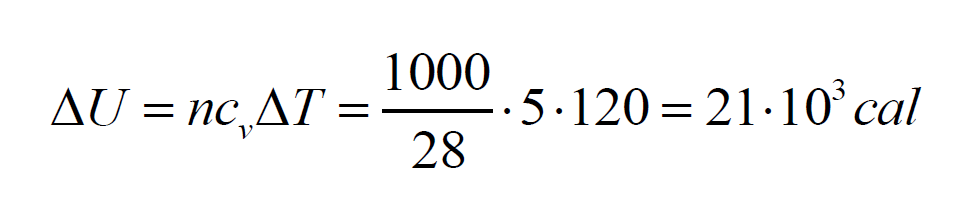c) The required external work:d) The required heat for a constant volume is: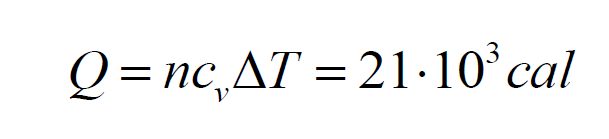We hope that you like this work equation. Thermodynamics is not easy to study, and this sample can help to make it easier. It was created to allow students to not miss anything while dealing with the equation. You can use this heat transfer formula. Thermodynamics assignments will be done quicker if you have the right samples like this one.